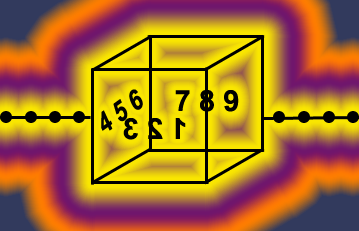2.2 - Dimension of "Non-Fractals"HandsOn Activities:

6. 1-, 2-, and 3-Dimensions

7. Circle Measurement of Dimension

We have been measuring the non-integer dimension of coastlines. The shape of a coastline is called a fractal. Non-fractal objects have integer dimensions. For example, a line is 1-dimensional. A non-crumpled piece of paper is 2-dimensional. A cube is 3-dimensional, as shown in Figure 2.6.

Previous: SimuLab 2 - Covering a Coastline